# QuTiP lecture: Jaynes-Cummings-like model in the ultrastrong coupling regime¶

Author: J. R. Johansson ([email protected]), http://dml.riken.jp/~rob/

The latest version of this IPython notebook lecture is available at http://github.com/jrjohansson/qutip-lectures.

The other notebooks in this lecture series are indexed at http://jrjohansson.github.com.

In :
# setup the matplotlib graphics library and configure it to show figures inline in the notebook
%matplotlib inline
import matplotlib.pyplot as plt
import numpy as np

In :
# make qutip available in the rest of the notebook
from qutip import *


# Introduction¶

In the Jaynes-Cumming model, the dipole-interaction term between the atom and the cavity field is assumed to be weak, so that a rotating-wave approximation can be performed. For large coupling strengths between the atom and the cavity field the RWA is not justified, and for very large coupling strength interesting properties of the atom-cavity ground state is observed.

To explore this using QuTiP, consider the Hamiltonian

### $H = \hbar \omega_c a^\dagger a + \frac{1}{2}\hbar\omega_a\sigma_z + \hbar g(a^\dagger + a)(\sigma_- + \sigma_+)$.¶

Note that here we have not transformed the interaction part of the Hamiltonian using the RWA, for which the Hamiltonian would have been

### $H_{\rm RWA} = \hbar \omega_c a^\dagger a + \frac{1}{2}\hbar\omega_a\sigma_z + \hbar g(a^\dagger\sigma_- + a\sigma_+)$.¶

In this notebook we will calculate the ground state of the Hamiltonian $H$ as a function of the interaction strength $g$ (try to set use_rwa = True to use $H_{\rm RWA}$ instead).

The regime $g$ is large compared with all other energy scales in the Hamiltonian $H$ is called the ultrastrong coupling regime, and has been an active topic of research in recent years. See references below.

References:

### Problem parameters¶

Here we use units where $\hbar = 1$:

In :
wc = 1.0  * 2 * pi  # cavity frequency
wa = 1.0  * 2 * pi  # atom frequency

N = 15              # number of cavity fock states
use_rwa = False


### Setup the operators and the Hamiltonian¶

In :
# operators
a  = tensor(destroy(N), qeye(2))
sm = tensor(qeye(N), destroy(2))

na = sm.dag() * sm  # atom
nc = a.dag() * a    # cavity

# decoupled Hamiltonian
H0 = wc * a.dag() * a + wa * sm.dag() * sm

# interaction Hamiltonian
if use_rwa:
H1 = (a.dag() * sm + a * sm.dag())
else:
H1 = (a.dag() + a) * (sm + sm.dag())


## Find ground state as a function of coupling strength¶

In :
g_vec = np.linspace(0, 2.0, 101) * 2 * pi # coupling strength vector

psi_list = []

for g in g_vec:

H = H0 + g * H1

# find the groundstate and its energy
gnd_energy, gnd_state = H.groundstate()

# store the ground state
psi_list.append(gnd_state)


Calculate the cavity and atom excitation probabilities as for the calculated ground states:

In :
na_expt = expect(na, psi_list) # qubit  occupation probability
nc_expt = expect(nc, psi_list) # cavity occupation probability


Plot the ground state occupation probabilities of the cavity and the atom as a function of coupling strenght. Note that for large coupling strength (the ultrastrong coupling regime, where $g > \omega_a,\omega_c$), the ground state has both photonic and atomic excitations.

In :
fig, axes = plt.subplots(1, 1, sharex=True, figsize=(8,4))

axes.plot(g_vec/(2*pi), nc_expt, 'r', linewidth=2, label="cavity")
axes.plot(g_vec/(2*pi), na_expt, 'b', linewidth=2, label="atom")
axes.set_ylabel("Occupation probability", fontsize=16)
axes.set_xlabel("coupling strenght", fontsize=16)
axes.legend(loc=0)

fig.tight_layout()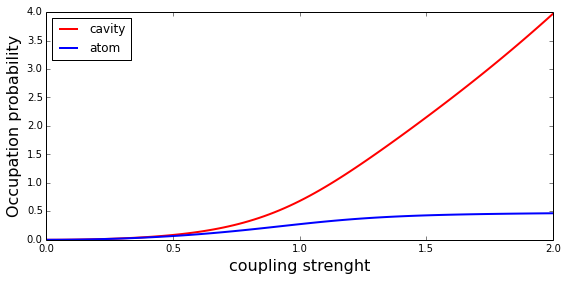# Plot the wigner functions of the cavity as a function of coupling strength¶

In :
g_idx = where([g_vec == 2*pi*g for g in [0.0, 0.5, 1.0, 1.5, 2.0]])
psi_sublist = array(psi_list)[g_idx]

xvec = np.linspace(-5,5,200)

fig_grid = (2, len(psi_sublist)*2)
fig = plt.figure(figsize=(3*len(psi_sublist),6))

for idx, psi in enumerate(psi_sublist):
rho_cavity = ptrace(psi, 0)
W = wigner(rho_cavity, xvec, xvec)
ax = plt.subplot2grid(fig_grid, (0, 2*idx), colspan=2)
ax.contourf(xvec, xvec, W, 100, norm=mpl.colors.Normalize(-.125,.125), cmap=plt.get_cmap('RdBu'))
ax.set_title(r"$g = %.1f$" % (g_vec[g_idx][idx]/(2*pi)), fontsize=16)

# plot the cavity occupation probability in the ground state
ax = plt.subplot2grid(fig_grid, (1, 1), colspan=(fig_grid-2))
ax.plot(g_vec/(2*pi), nc_expt, label="Cavity")
ax.plot(g_vec/(2*pi), na_expt, label="Atom excited state")
ax.legend(loc=0)
ax.set_xlabel('coupling strength')
ax.set_ylabel('Occupation probability')

Out:
<matplotlib.text.Text at 0x7ffb5a37bba8>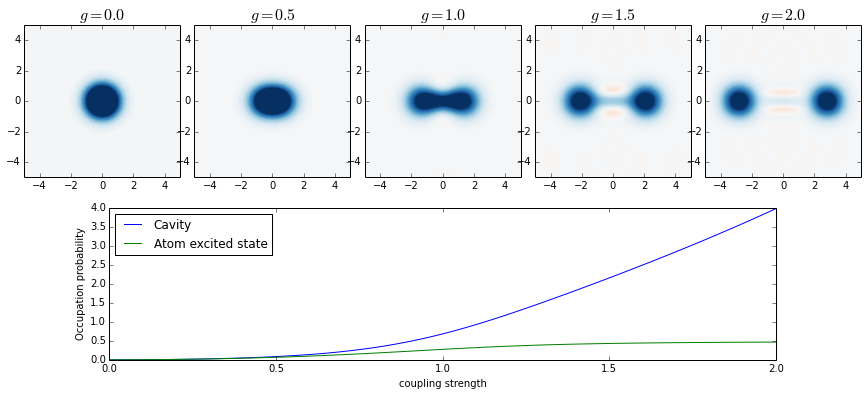## Entropy of atom/cavity as a measure of entanglement¶

In :
entropy_cavity = zeros(shape(g_vec))
entropy_atom   = zeros(shape(g_vec))

for idx, psi in enumerate(psi_list):

rho_cavity = ptrace(psi, 0)
entropy_cavity[idx] = entropy_vn(rho_cavity, 2)

rho_atom = ptrace(psi, 1)
entropy_atom[idx]   = entropy_vn(rho_atom, 2)

In :
fig, axes = plt.subplots(1, 1, figsize=(12,6))
axes.plot(g_vec/(2*pi), entropy_cavity, 'b', label="cavity", linewidth=2)
axes.plot(g_vec/(2*pi), entropy_atom, 'r--', label="atom", linewidth=2)
axes.set_ylim(0,1)
axes.set_ylabel("entropy", fontsize=16)
axes.set_xlabel("coupling strength", fontsize=16)
axes.legend(loc=0)

Out:
<matplotlib.legend.Legend at 0x7ffb5a2002b0>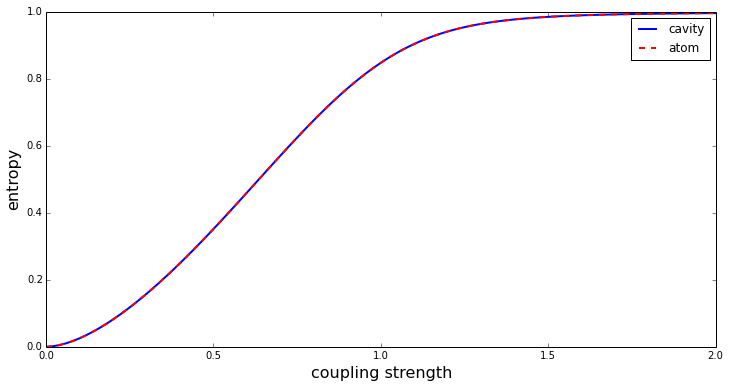## Dynamics of an initially excited cavity¶

In :
H = H0 + 1.0 * 2 * pi * H1

psi0 = tensor(basis(N,1), basis(2,0))

In :
tlist = np.linspace(0, 20, 1000)
output = mesolve(H, psi0, tlist, [], [a.dag() * a, sm.dag() * sm])

In :
fig, axes = plt.subplots(1, 1, sharex=True, figsize=(8,4))

axes.plot(tlist, real(output.expect), 'r', linewidth=2, label="cavity")
axes.plot(tlist, real(output.expect), 'b', linewidth=2, label="atom")
axes.legend(loc=0)

fig.tight_layout()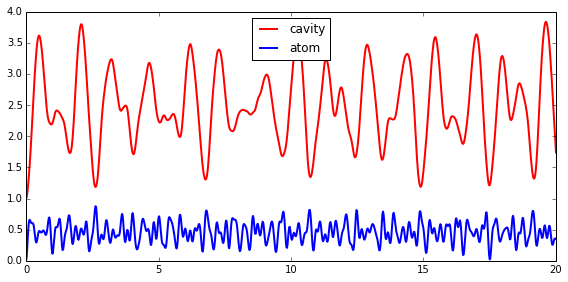### Fock-state distribution and Wigner function for the cavity as a function of time¶

In :
tlist = np.linspace(0, 0.35, 8)
output = mesolve(H, psi0, tlist, [], [])

In :
rho_ss_sublist = output.states #[::4]

xvec = np.linspace(-5,5,200)

fig, axes = plt.subplots(2, len(rho_ss_sublist), figsize=(2*len(rho_ss_sublist), 4))

for idx, rho_ss in enumerate(rho_ss_sublist):

# trace out the cavity density matrix
rho_ss_cavity = ptrace(rho_ss, 0)

# calculate its wigner function
W = wigner(rho_ss_cavity, xvec, xvec)

# plot its wigner function
axes[0,idx].contourf(xvec, xvec, W, 100, norm=mpl.colors.Normalize(-.25,.25),
cmap=plt.get_cmap('RdBu'))

# plot its fock-state distribution
axes[1,idx].bar(arange(0, N), real(rho_ss_cavity.diag()), color="blue", alpha=0.6)
axes[1,idx].set_ylim(0, 1)
axes[1,idx].set_xlim(0, N)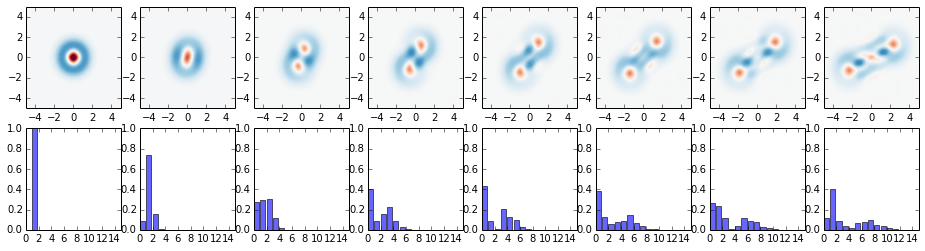### Same thing with a little bit of dissipation¶

In :
kappa = 0.25

In :
tlist = np.linspace(0, 20, 1000)
output = mesolve(H, psi0, tlist, [sqrt(kappa) * a], [a.dag() * a, sm.dag() * sm])

In :
fig, axes = plt.subplots(1, 1, sharex=True, figsize=(8,4))
axes.plot(tlist, output.expect, 'r', linewidth=2, label="cavity")
axes.plot(tlist, output.expect, 'b', linewidth=2, label="atom")
axes.legend(loc=0)

Out:
<matplotlib.legend.Legend at 0x7ffb594addd8>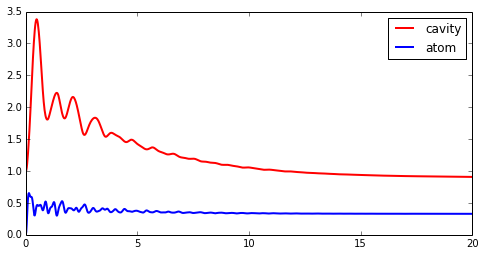In :
tlist = np.linspace(0, 10, 8)
output = mesolve(H, psi0, tlist, [sqrt(kappa) * a], [])

In :
xvec = np.linspace(-5,5,200)

fig, axes = plt.subplots(2, len(output.states), figsize=(2*len(output.states), 4))

for idx, rho_ss in enumerate(output.states):

# trace out the cavity density matrix
rho_ss_cavity = ptrace(rho_ss, 0)

# calculate its wigner function
W = wigner(rho_ss_cavity, xvec, xvec)

# plot its wigner function
axes[0,idx].contourf(xvec, xvec, W, 100,
norm=mpl.colors.Normalize(-.25,.25), cmap=plt.get_cmap('RdBu'))

# plot its fock-state distribution
axes[1,idx].bar(arange(0, N), real(rho_ss_cavity.diag()), color="blue", alpha=0.6)
axes[1,idx].set_ylim(0, 1)
axes[1,idx].set_xlim(0, N)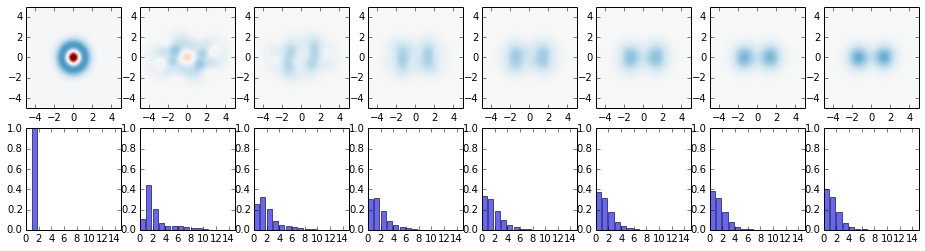### Entropy as a function of time in presence of dissipation and starting in the ideal ground state¶

In :
tlist = np.linspace(0, 30, 50)

psi0 = H.groundstate()

output = mesolve(H, psi0, tlist, [sqrt(kappa) * a], [])

In :
entropy_tot    = zeros(shape(tlist))
entropy_cavity = zeros(shape(tlist))
entropy_atom   = zeros(shape(tlist))

for idx, rho in enumerate(output.states):

entropy_tot[idx] = entropy_vn(rho, 2)

rho_cavity = ptrace(rho, 0)
entropy_cavity[idx] = entropy_vn(rho_cavity, 2)

rho_atom = ptrace(rho, 1)
entropy_atom[idx]   = entropy_vn(rho_atom, 2)

In :
fig, axes = plt.subplots(1, 1, figsize=(12,6))
axes.plot(tlist, entropy_tot, 'k', label="total", linewidth=2)
axes.plot(tlist, entropy_cavity, 'b', label="cavity", linewidth=2)
axes.plot(tlist, entropy_atom, 'r--', label="atom", linewidth=2)
axes.set_ylabel("entropy", fontsize=16)
axes.set_xlabel("coupling strength", fontsize=16)
axes.set_ylim(0, 1.5)
axes.legend(loc=0)

Out:
<matplotlib.legend.Legend at 0x7ffb59799c88>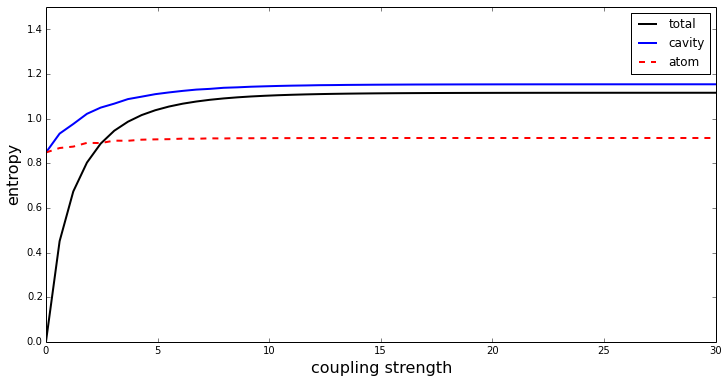### Software versions¶

In :
from qutip.ipynbtools import version_table

version_table()

Out:
SoftwareVersion
QuTiP3.0.0.dev-5a88aa8
matplotlib1.3.1
Numpy1.8.1
Cython0.20.1post0
SciPy0.13.3
OSposix [linux]
IPython2.0.0
Python3.4.1 (default, Jun 9 2014, 17:34:49) [GCC 4.8.3]
Thu Jun 26 14:32:43 2014 JST